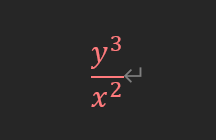# How to reformulate this in a CVX way: Cube over Square

How to reformulate this in a CVX way. I know it doesn’t meet the DCP ruleset, but is there any method to reformulate this expression?
x>0, y>0My `z` is your `y`, and my `y` is your `x`.

Thank you! Mark! Yes, I just found this answer in previous topics and thank you again!

Sorry, Mr. Mark, I still have a question that is there difference when using this solution in the object function instead of constraints?

You do the same thing whether in objective function or constraints.

In my solution, use x in place of z^3/y^2 wherever it appears . Change `x` to whatever variable name you have chosen to serve its role.

Thank you, I will try. It is so nice to have your help！ Thank you, Mark!

For educational purposes, there is an alternative way of doing this with geometric mean (`geo_mean`), as suggested by @Erling in Disciplined convex programming error: Illegal operation: pow_p( {convex}, {-1} )

Hi Mark~Thank you for this solution. When I used the rotated_lorentz method, it reported something wrong like “Invalid constraint: {convex} == {real affine}”
My code is like: (Dm is your z and Tm is your y), and I used the “for” loop to generate this M dimension vector. Could you please help figure out why this is wrong?
for i=1:M
{Dm(i),s_temp(i),Tm(i)} == rotated_lorentz(1);
{s_temp(i),x_temp(i),Dm(i)} == rotated_lorentz(1);
end

All the arguments inside `{}` must be affine (which can be a variable). if your `y` and `x` are CVX variables or affine expressions, this should be o.k. Otherwise, it is not o.k., and might not necessarily be convex. Based on the error message, one or more of the arguments is convex, but not affine.

My expression Dm and variables Tm are defined as:
variables qm(M+1,2) Tm(M,1)
variables x_temp(M,1) s_temp(M,1)
expression Dm(M,1)
for i=1:M
Dm(i) = norm(qm(i+1,:)-qm(i,:));
end

In my loop, the expression and variables are scalar and how these arguments are convex? Thank you again for your reply

`Dm is a norm, not an affine expression. Hence this will not work, and as far as I know, is not convex. Bit if someone knows otherwise, they are free to weight in.

The Dm is the distance variable which denotes the distance between two 2-D location variables . What do you mean “weight in”? Is this a method to transfer the convex to affine?

I meant someone can post why your problem is convex, if they have shown it is convex. Otherwise, I will assume it is not convex.

My prove process is like this.

So Dm is convex because sqrt(f) is convex and non-decreasing, and f is convex

Norm of an affine argument is convex, so `Dm` is convex. But the cube over square involving it, is not convex as far as I know.

So you mean that Dm^3/Tm^2 is not convex? How about Dm^2/Tm?

For `Dm^2/Tm`, you should be able to use `quad_over_lin`, in which case `norm` would never be explicitly used in the formulation.

For cube over square, @Erling 's approach, as linked in my earlier post, should work if one or both of the items being cubed or squared is concave (or affine), but would not work if either item is convex, which is your situation. That is because all argument of `geo_mean` mus be concave (or affine).

As the Dm is convex, the geo_mean couldn’t work. So, is there any way to express Dm^3/Tm^2 in cvx?

As far as I know, no. Please carefully read the "Why isn’t CVX accepting my model? READ THIS FIRST! " link provided above.

OK， thank you! I will check whether it is convex.